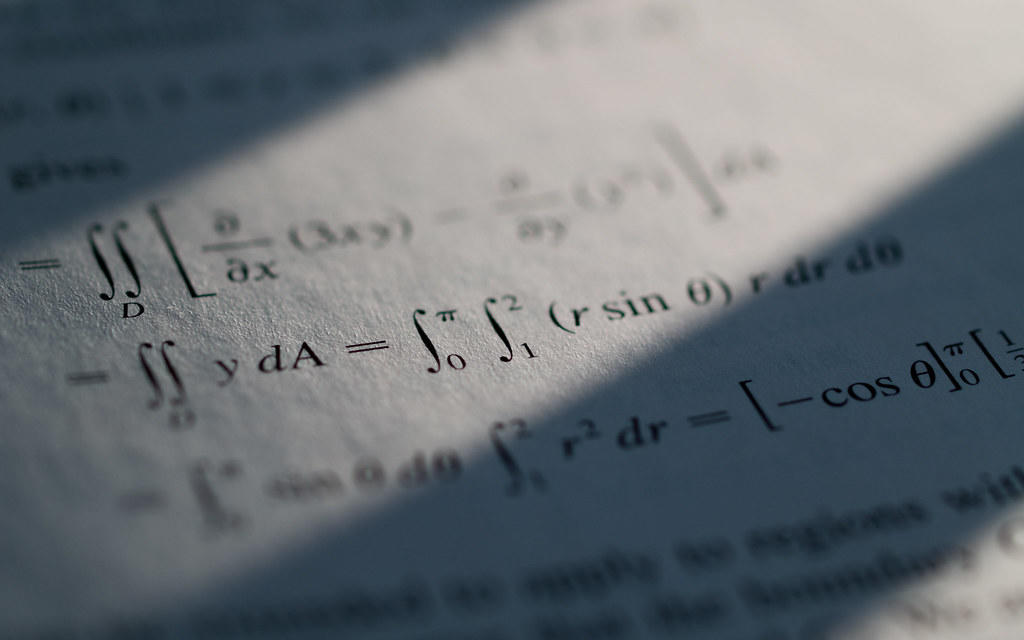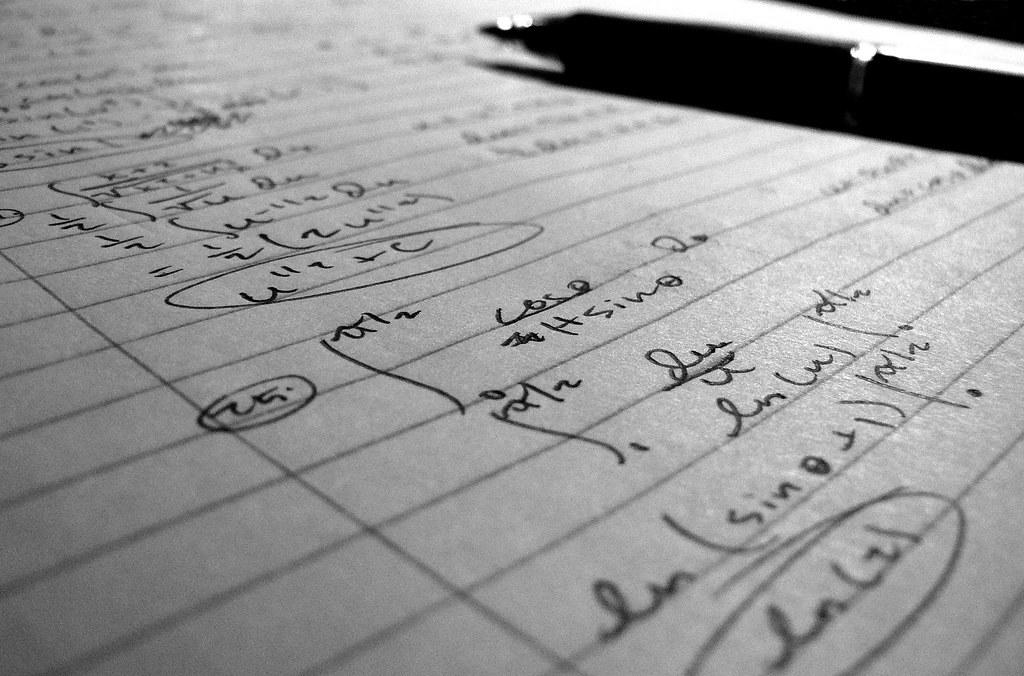There are several branches of mathematics, and calculus is one of them. Although considered complicated, one can define it simply as a study on rates of change.

Before calculus, math was static, meaning that only objects that were still could be calculated. However, since the universe is constantly shifting and moving, calculus became essential.

Its inception is justified by the realization that no object in the universe, not even the stars or our cells and atoms, is always at rest.

Therefore, the discipline was developed to determine the rate of change between matter, stars, and particles and how they moved.

Calculus, surprisingly, is not only limited to mathematicians. Several other fields use the concepts explored in calculus, such as medicine, physics, statistics, economics, and engineering.

It can also be used to understand space travel, build structures more securely, and determine the interactions of medicines with your body.

This article will discover everything to do with calculus: who invented it, how it is applied, and the best ways to learn it.The best Mathematics tutors available5 (54 reviews)
Davayne
\$13
/h1st lesson free!5 (25 reviews)
Jeffry
\$48
/h1st lesson free!4.9 (11 reviews)
Lyle
\$30
/h1st lesson free!5 (6 reviews)
Bryan
\$25
/h1st lesson free!4.9 (14 reviews)
Momin khan
\$10
/h1st lesson free!5 (11 reviews)
Anh
\$25
/h1st lesson free!5 (4 reviews)
Beccalynn
\$18
/h1st lesson free!5 (82 reviews)
Dr. Mary (professional expert tutor)
\$50
/h1st lesson free!5 (54 reviews)
Davayne
\$13
/h1st lesson free!5 (25 reviews)
Jeffry
\$48
/h1st lesson free!4.9 (11 reviews)
Lyle
\$30
/h1st lesson free!5 (6 reviews)
Bryan
\$25
/h1st lesson free!4.9 (14 reviews)
Momin khan
\$10
/h1st lesson free!5 (11 reviews)
Anh
\$25
/h1st lesson free!5 (4 reviews)
Beccalynn
\$18
/h1st lesson free!5 (82 reviews)
Dr. Mary (professional expert tutor)
\$50
/h1st lesson free!

## The Inventors of Calculus

The development of Calculus took place around the end of the 17th century. It was heralded by renowned mathematicians Isaac Newton and Gottfried Leibniz.

However, Newton was the first to develop calculus and apply it directly to understand how physical systems work.Isaac Newton was a mathematician and physicist who discovered gravity and also developed calculus. He is one of the most famous scientists from the 17th century, and because of him, we now have calculus bases (Source: Visual Hunt)

Leibniz was the one to develop the notions that are used within calculus. Simply put, basic math makes use of operations such as:

• Multiplication
• Division
• Subtraction

In contrast, calculus utilizes different operations to calculate rates of change, such as:

• Integrals
• Functions

The development of these tools allowed Leibniz and Newton, and other mathematicians to calculate, for example, the ‘exact slope of a curve at any point.’

Using calculus bases, astronomers, mathematicians, chemists, and scientists could now graph down the trajectory of stars and planets and note down the path of protons and electrons at an atomic level.

## The Practical Applications Of Calculus

Calculus is an interdisciplinary tool that is relied on quite heavily. Its value is realized by physicians, chemists, geographers, and engineers alike.

Calculus can be applied to several practical life situations. Some calculus concepts are very relevant to:

• Light
• Astronomy
• Acoustics
• Motion
• Heat
• Harmonics
• Electricity

Additionally, calculus is also used in other vital subjects such as:

• Robotics
• Computer vision
• Movies
• Video games
• Geography
• Artificial intelligence
• Photography

Here are some applications of chemistry across a spectrum of fields:

### Chemistry

Its usefulness in chemistry is highlighted when calculating:

• Radioactive decay rates to study planetary motions and gravity
• Ship design
• The prediction of death and birth rates
• Bridge engineering
• Geometric curves

### Physics

Calculus is used in physics to explain, calculate and define:

• Dynamics
• Heat
• Motion
• Harmonics
• Light
• Electricity
• Astronomy
• Acoustics

Moreover, the physics theory of relativity concocted by Albert Einstein heavily relies on calculus.

### Others

Economists also make use of calculus when predicting the profit-making capabilities of an industry or company.

And in shipbuilding, differential calculus is used to determine the hull and curve of the ship, whereas integral calculus is used to calculate the area underneath the hull. It is also generally used when ships are being designed.

And lastly, different disciplines of math also use calculus to check answers, such as in analytical geometry, algebra, and statistics.

## 8 Of The Best Ways To Effectively Learn Calculus

As of 2018, stats showed that 800,000 students all over the US were taking calculus at the high school level, which amounts to 15% of the total number of high schoolers.

And what is underwhelming is that only 150,000 were taking the calculus course before reaching the 11th grade.

Although it consists of concepts outside of mathematics, it is still a branch of it. Some of the main functions utilized in calculus are:

• Functions
• Limits
• Integrals
• Derivatives

This is why many people feel that the subject is complex. However, it is a significant part of the math family and may also be the most complicated subject because students struggle with it immensely.

However, calculus can be easily understood if students go about the learning process in the right way. Here are some of the steps students can follow to understand calculus and score good marks in it:

### 1. Start By Studying Other Mathematical ComponentsCalculus was initially known as “the calculus of infinitesimals” or infinitesimal calculus. This is because it is a mathematical study of objects that are continuously changing. For a better understanding of calculus concepts, hire a private calculus tutor. (Source: Visual Hunt)

Calculus is a field of math that is considered to be linked to many other mathematical aspects.

Therefore, if students work on those before delving into math, they will better understand the discipline. The essential mathematical components used in calculus are:

• Geometry: You should familiarize yourself with the properties and shapes related to it
• Algebra: Study the different properties of algebra and get a clear understanding of groups and sets
• Arithmetic: You should understand basic arithmetic and be able to apply all its different operations

### 2. Know Which Parts Are Relevant to Calculus

Calculus as a whole is further divided into two categories:

• Integral calculus
• Differential calculus

The rate of change is a derivative, while accumulation is integral. Calculus is primarily related to rate, so you should devote time to understanding the rate of change time vs. distance, time vs. velocity, etc.

### 3. Learn Different Calculus Formulas

The integrals and derivatives that form calculus are determined using a basic formula that you will need to memorize before you can solve calculus problems.

Do not focus on just memorizing it, but instead, take the time to understand how the formulae are used by looking at examples of calculus equations.

### 4. Learn About The Concept Of Limits

To evaluate a complex function, you will need to break it down into smaller pieces, which is done through ‘limits.’

Solve the complex function while it is fragmented and then put it back together. This makes it easier to solve it; hence, understanding limits and how to use them is critical.

### 5. Understanding The Fundamental Theorem Of Calculus

As dull as it may sound, you cannot skip studying the fundamental theorem of calculus if you want to understand it properly.

This theorem is the basis through which students understand differentiation and integration. It also helps them understand how these two are usually inverse of one another.

### 6. Practice Calculus Problems

Once you have taken the time to understand the theorem and formulae, you can start practicing calculus problems.

First, you should focus on examples of calculus problems, ideally, those involving derivatives. Once you have the hang of those, you can move on to integral issues.During the learning process, it is vital to take a look at examples of calculus problems to get a better idea of what they are like. The more comfortable and less intimidated you are while attempting them, the better! (Source: Visual Hunt)

To become adept at calculus, you must practice as many problems as possible every single day. If you are stuck at any point, discuss it with a teacher, peers, or a private calculus tutor who can take out the time to explain any complicated concepts calmly. Then, proceed and keep practicing.

### 7. Make A Note Of The Troubles You Are Facing

Of course, you are not expected to go smooth sailing when learning calculus. You learn every step of the way, and many students remain confused regarding calculus formulae, problems, or theorem.

In most cases, they would be happy to help you. However, if you feel uncomfortable asking questions in a class setting, you can always refer to a private calculus tutor.

If you wonder how to find a calculus tutor, you can visit Superprof’s site to find hundreds of experienced calculus teachers within any budget you have.

### 8. Go Through Your Concepts Once More

Even if you have learned the different calculus concepts and have gotten into the flow of answering related questions, you can still continually improve. It would help if you considered going through the various concepts again to gauge whether you have a proper understanding of all the concepts or not.

It is better to clear any doubts you have after understanding the subject to better navigate calculus problems.

Even though calculus is a relatively tricky math subject, it is an essential branch of it. So, even if you are currently struggling or embarking on your calculus journey, you can follow these eight steps to grasp the subject better and get the desired results.

## Have Fun Learning Calculus!

Calculus is a mathematics-related subject that students either love or hate. On the one hand, it can expose you to the practical beauty of math, whereas, on the other hand, it can represent frustration!

Some calculus concepts can be mind-bending, but as discussed, there are many ways you can work through the learning process.

Whether you use online resources or a private tutor, remember that there is a way when there is a will. However, we recommend the latter.

Most Superprof teachers offer the first lesson for free, and perhaps the best part is that you can look at how much each charges and choose one within your budget!The platform that connects tutors and students5.00 (1 rating(s))Loading...